## 1. Introduction

Let’s imagine that we have a set of points on aplane that we’d like to connect to draw a closed shape. First, we’d need to sort those points.

In our tutorial, we’re going to walk through a method that can sortpoints in clockwise order.

## 2. Problem Explanation

Given a set of 2D points (each represented byandcoordinates), we would like to sort these points in clockwise order. To begin, we need to view these points around a center point. This center-point can be at thecoordinates, or it could be at any arbitrary point on the 2D plane. Based on the requirements, we can choose which center point is better.

In our scenario, we’ll estimate the center point as the mean of the given points. This is shown in the following figure where we have 3 points sorted around the center point: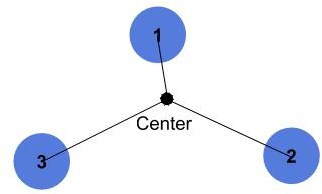There are a few points to note here. First, we aren’t estimating the convex hull (the convex that encapsulates all points), but we’d like to find a closed path that goes through all the points in a clockwise order. Thus, it is fine to sort some points in the following way: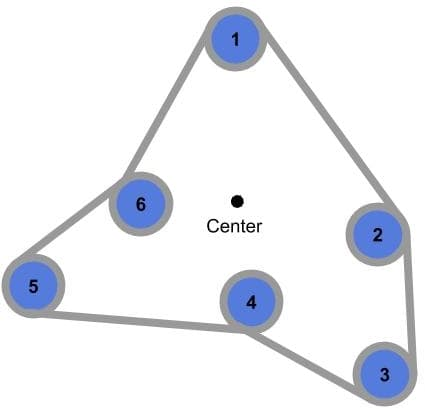However, for the same set of points, if we estimate the convex hull, we’ll only include points 1, 2, 3, and 5 as follows: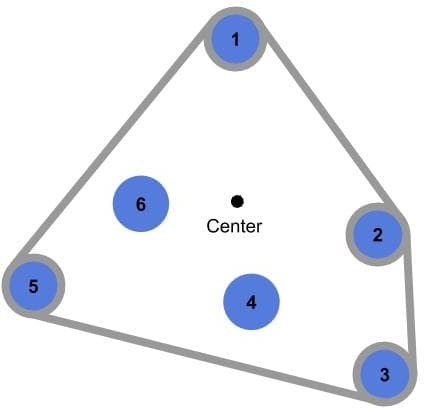One last thing to note is that we aren’t going to cover the exceptional case where all points lie on a straight line.

## 3. Algorithm Idea

Let’s walk through an example given the set of points,,,,, andas shown: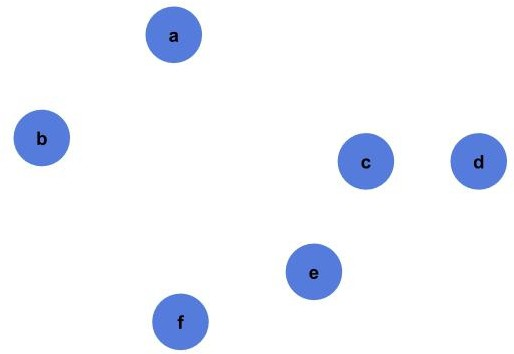The first thing we need to do is to find the center point of the given points: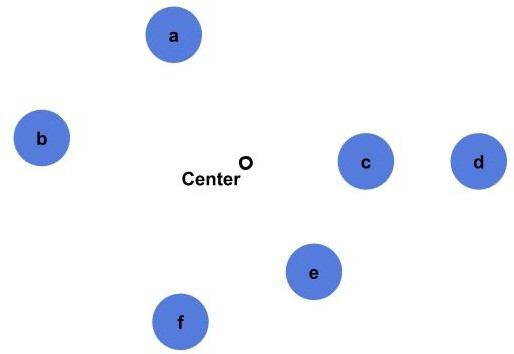Then we estimate the angle between each point and the center: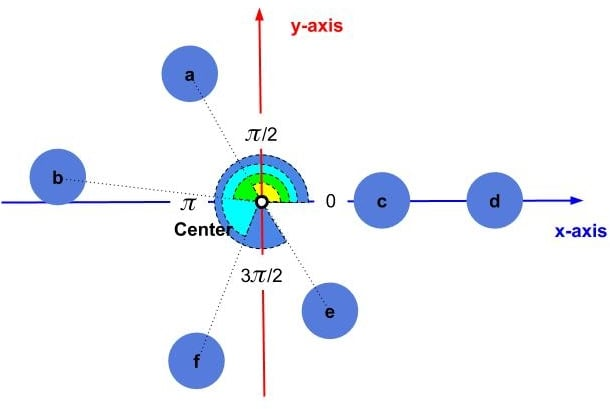Since we need to sort the points in clockwise order, the first point will be pointas it falls in thequadrant. Next will be pointas it falls in thequadrant. Pointsandare in thequadrant; however,comes first as it is closer to, andis next. Finally, we have pointsandin thequadrant, but both are at angle. In this tutorial, we decided to break the tie by the distance from the center: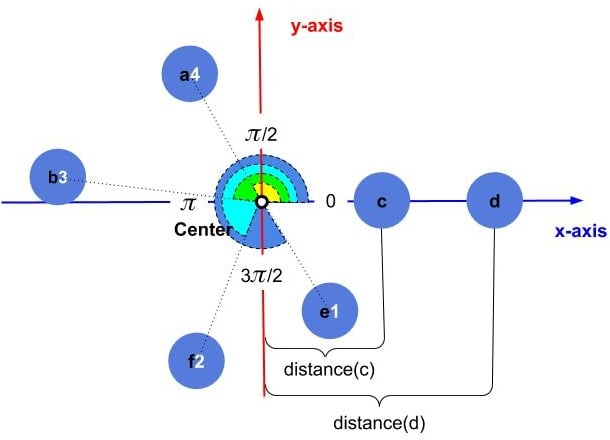Since pointis closer to the center than point,becomes thepoint, andis the last point in our order: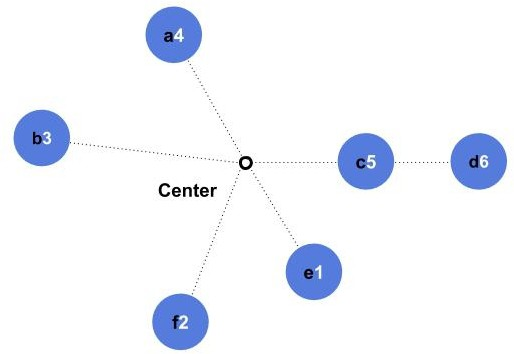The final order in the given example will be,,,,,.

## 4. Flow-Chart

Now let’s see more details on how to design the algorithm.

From a high-level perspective, we have an array of points that we need to sort according to some criteria. We can use any sorting algorithm and provide the right comparator to do the job; however, in our case, we need to add some pre- and post-processing steps.

For the pre-processing steps, we need to loop over the points to get the center point. Then we subtract this center point from all the given points so that we translate all the points to the new origin (center point).

Next, we call our sorting algorithm (like Quicksort or Bubble-Sort) with ourfunction to get the points sorted according to our defined criteria. Finally, we re-add the center point to the sorted points to translate them back to the original location.

### 4.1. Sort Points Flow-Chart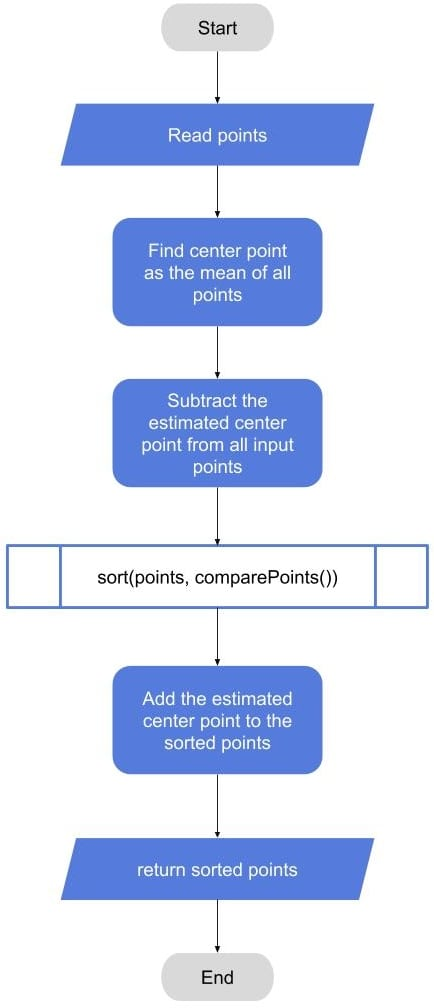### 4.2. Compare Points Flow-Chart

Now let’s dig deeper inside thefunction. In this function, we get two points as inputs, and we need to returnif the first point comes before the second point, andotherwise. If we assume we have another function telling us the angle of any point relative to some center point fromto, then we can compare the two angles directly. In the case of a tie, we can compare the distance between each point and the center point, and then choose the smallest one.

The following flow-chart illustrates this idea. The center point isbecause we translated all of our points around the center point in the pre-processing step: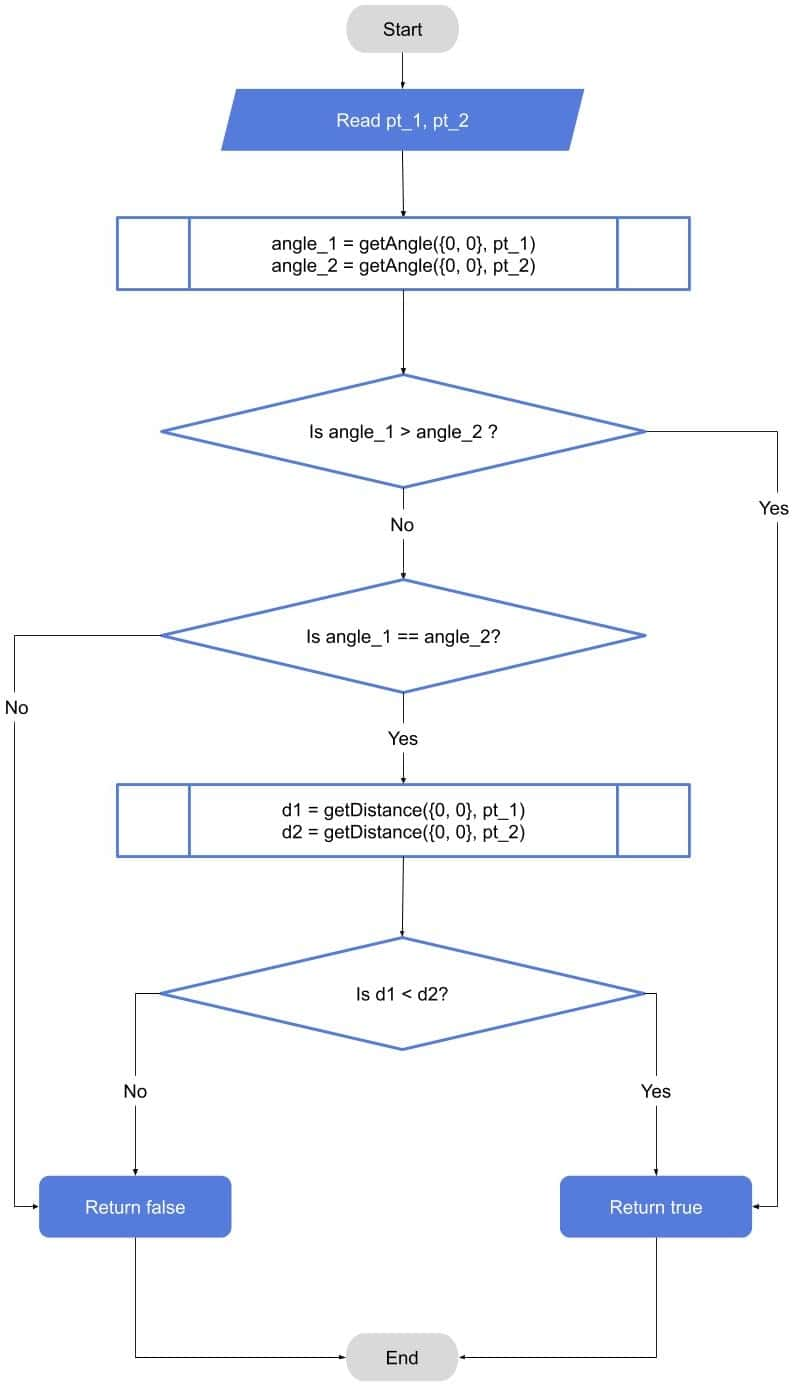The details for theandfunctions are going to be in the pseudocode.

## 5. Pseudocode

Now let’s look at simple pseudocode for our algorithm. The pseudocode starts with the general algorithm of finding the center point, and then translates all the points to this center. Next we call the sort function with our customfunction. Finally, we move the sorted points back to the original locations:Ourfunction then calls theandfunctions for each pair of points. The function returnsin the cases where the first angle is bigger than the second angle. In the cases where the angles are equal, or the first distance is less than the second distance:Now let’s see how to estimate the angle. We’re going to assume that we’re using a function likethat exists in many programming languages. This function estimates the angle using inverse tan based on the value ofand, and returns the result in the rangeto. In our case, we need the angle to be betweenand. One way to do this is if the angle is less than, we add. Thus, the final value of the angle will be in the desired range fromtoAs for the distance, we can use simple Euclidean distance as the square root () of the sum of the square of the differences for all axes. In practice, we can get rid of the square root step and return the squared distance (in our scenario the distance is always between some point and the center point):Please note that the sorting algorithm itself is out of the scope of this tutorial. We can use any available sorting algorithm like Quicksort to get a complexity of.

## 6. Complexity

The time complexity of our algorithm is the complexity of the selected sorting algorithm. If we choose Quicksort, the overall algorithm complexity will be. The reason is that we only have loops of complexityfor pre- and post-processing. Then we call the sorting algorithm with our custom compare function.

As for the space complexity, the algorithm doesn’t need to store any information apart from the center point. Therefore, the space complexity is.

## 7. Conclusion

In this article, we presented a method to sort points in clockwise order. The idea depends on any general sorting algorithm with some pre- and post-processing steps. We illustrated the algorithm through an example, flow-charts, and pseudocode. Finally, we provided a simple complexity analysis of space and time complexities for the presented method.